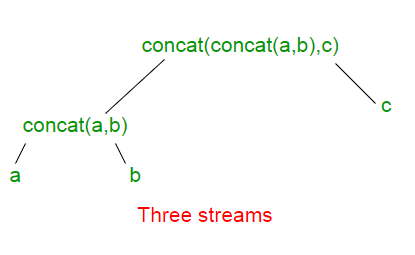# IntStream concat() in Java

• Last Updated : 06 Dec, 2018

IntStream concat() method creates a concatenated stream in which the elements are all the elements of the first stream followed by all the elements of the second stream. The resulting stream is ordered if both of the input streams are ordered, and parallel if either of the input streams is parallel.

Syntax :

```static IntStream concat(IntStream a,  IntStream b)

Where, IntStream is a sequence of primitive int-valued elements,
a represents the first stream,
b represents the second stream and
the function returns the concatenation of
the two input IntStreams.
```

The calls to IntStream.concat(IntStream a, IntStream b) can be think of as forming a binary tree. The concatenation of all the input streams is at the root. The individual input streams are at the leaves. Below given is example for 3 IntStreams a, b and c.Each additional input stream adds one layer of depth to the tree and one layer of indirection to reach all the other streams.

Note : The elements returned by IntStream.concat() method is ordered. For example, the following two lines returns the same result:

```IntStream.concat(IntStream.concat(stream1, stream2), stream3);
IntStream.concat(stream1, IntStream.concat(stream2, stream3));
```

But the result for the following two are different.

```IntStream.concat(IntStream.concat(stream1, stream2), stream3);
IntStream.concat(IntStream.concat(stream2, stream1), stream3);
```

Example 1 :

 `// Implementation of IntStream.concat()``// method in Java 8 with 2 IntStreams``import` `java.util.*;``import` `java.util.stream.IntStream;``import` `java.util.stream.Stream;`` ` `class` `GFG {`` ` `    ``// Driver code``    ``public` `static` `void` `main(String[] args)``    ``{``        ``// Creating two IntStreams``        ``IntStream stream1 = IntStream.of(``2``, ``4``, ``6``);``        ``IntStream stream2 = IntStream.of(``1``, ``3``, ``5``);`` ` `        ``// concatenating both the Streams``        ``// with IntStream.concat() function``        ``// and displaying the result``        ``IntStream.concat(stream1, stream2)``            ``.forEach(element -> System.out.println(element));``    ``}``}`

Output:

```2
4
6
1
3
5
```

Example 2 :

 `// Implementation of IntStream.concat()``// method in Java 8 with 2 IntStreams``import` `java.util.*;``import` `java.util.stream.IntStream;``import` `java.util.stream.Stream;`` ` `class` `GFG {`` ` `    ``// Driver code``    ``public` `static` `void` `main(String[] args)``    ``{``        ``// Creating two IntStreams``        ``IntStream stream1 = IntStream.of(``2``, ``4``, ``6``);``        ``IntStream stream2 = IntStream.of(``1``, ``2``, ``4``);`` ` `        ``// concatenating both the Streams``        ``// with IntStream.concat() function``        ``// and displaying distinct elements``        ``// in the concatenated IntStream``        ``IntStream.concat(stream1, stream2).distinct().``        ``forEach(element -> System.out.println(element));``    ``}``}`

Output:

```2
4
6
1
```

My Personal Notes arrow_drop_up